# The Rössler equations

The purpose of this demonstration is to show you how to produce all the figures in Strogatz's discussion of the Rössler system in Section 10.6 of the book. This uses material that I discussed in the earlier tutorials on function handles and ode45. It also makes use of a new concept in MATLAB's ODE solver, the events location feature, which will allow us to compute Poincaré sections.
The Rossler equations (Strogatz p 383) depend on three parameters a, b, c.The function ode45 requires that the function only take the two inputs t and y. The best way to pass the parameters is to use a MATLAB programming construct called anonymous functions, which is described in a separate document. The following example shows how to do this.
a=0.2; b=0.2; c=3.5;
myODE=@(t,y)rossler(t,y,a,b,c);
tspan=[0 100]; % Time span
y0=[1 0 0];
[t,y]=ode45(myODE,tspan,y0);
plot(t,y);legend('x(t)','y(t)','z(t)');xlabel('t')
title('Period-2 limit cycle with $c=3.5$')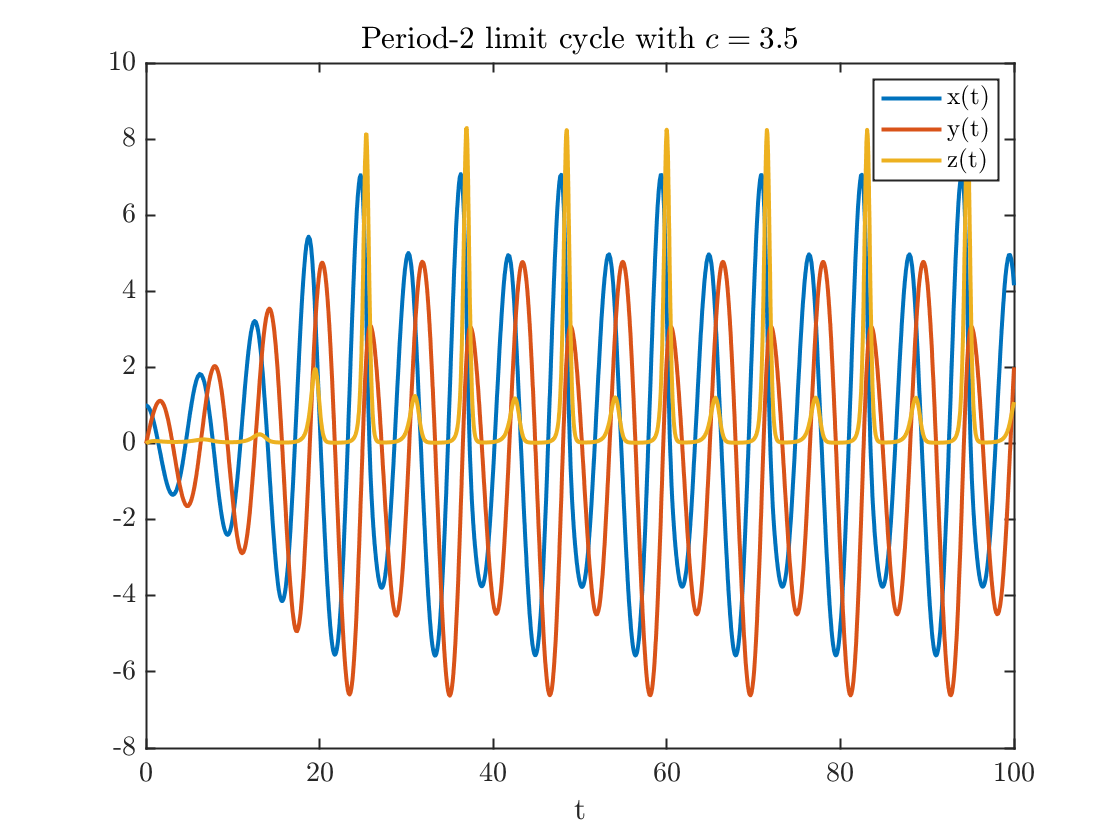figure
plot3(y(:,1),y(:,2),y(:,3))
xlabel('x');ylabel('y');zlabel('z')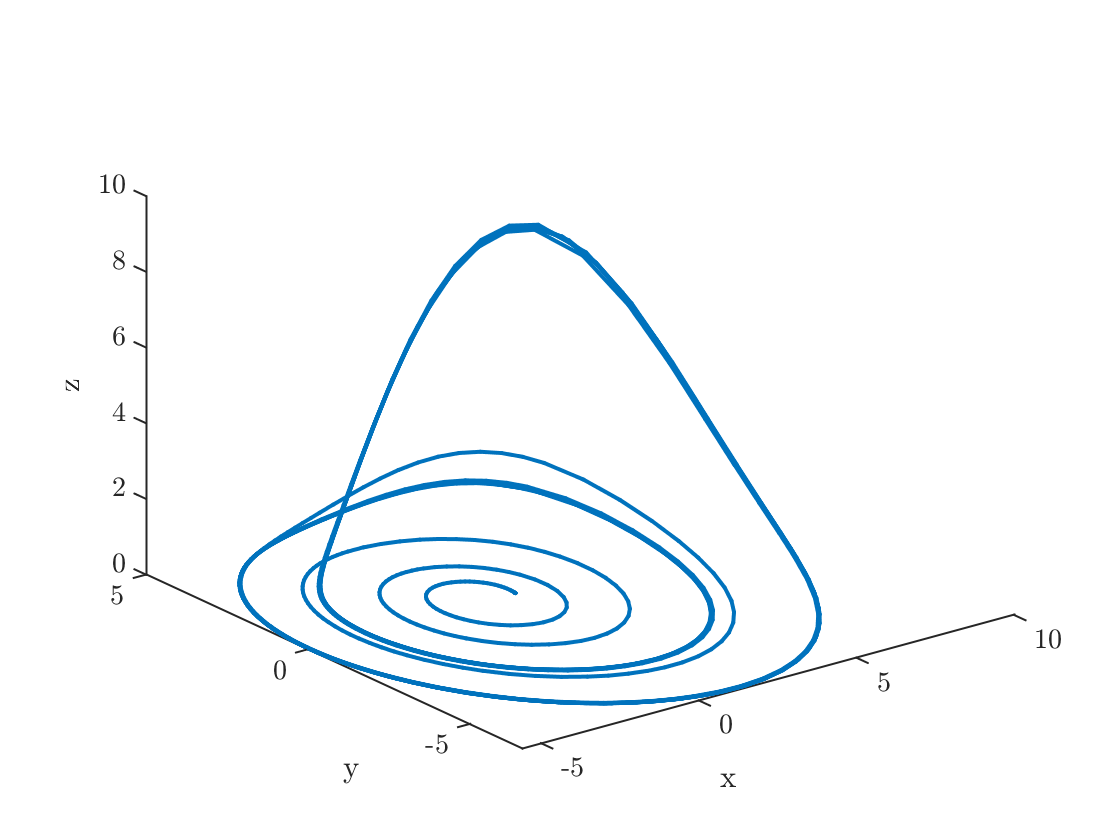The command myODE=@(t,y)rossler(t,y,a,b,c) defines a function handle to a function myODE that depends only on the two variables t and y. Note that since myODE is already a function handle, it does not need an @ sign.

## Four representative solutions

Reproducing Figure 10.6.6
cVector=[2.5 3.5 4 5];
for k=1:4
c=cVector(k);
myODE=@(t,y)rossler(t,y,a,b,c);
tspan=[0 600]; % Time span
tTransient=200;
y0=[1 0 0];
[t,y]=ode45(myODE,tspan,y0);
spot=find(t>tTransient,1,'first');
y=y(spot:end,:);
figure
plot(y(:,1),y(:,2))
axis equal square
xlabel('x');ylabel('y');
title(sprintf('$c=%0.1f$',c))
end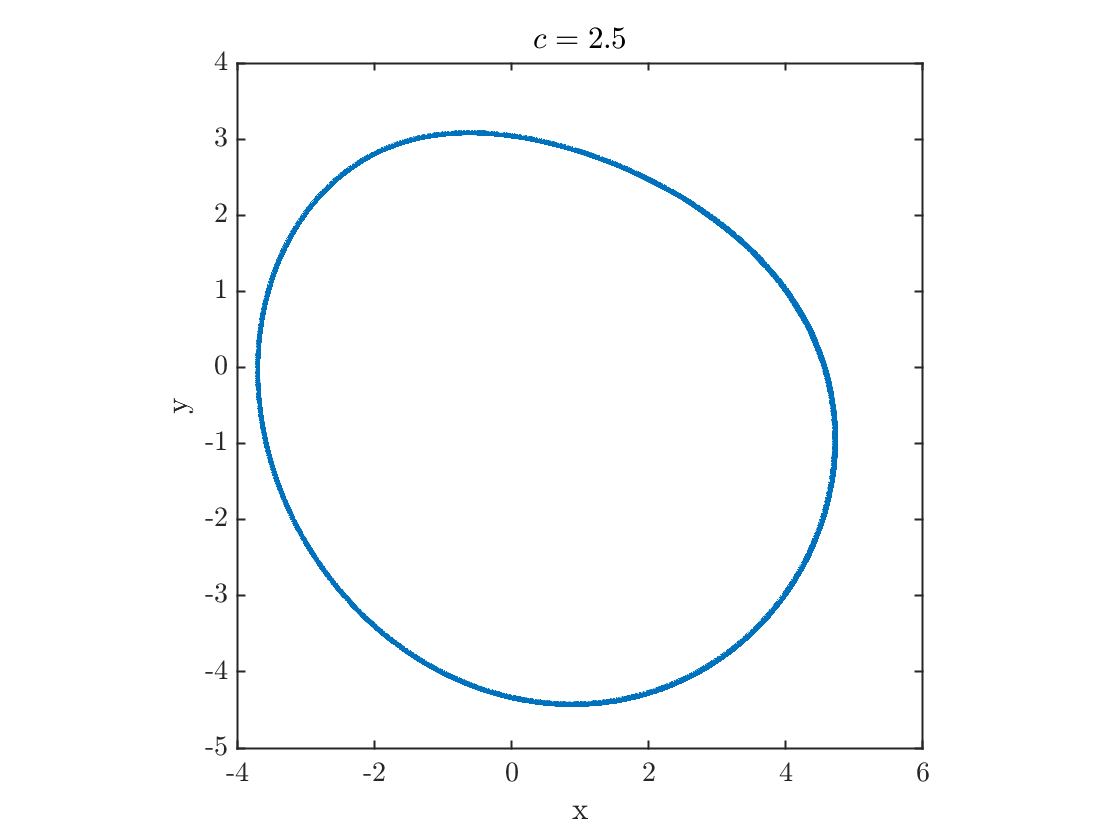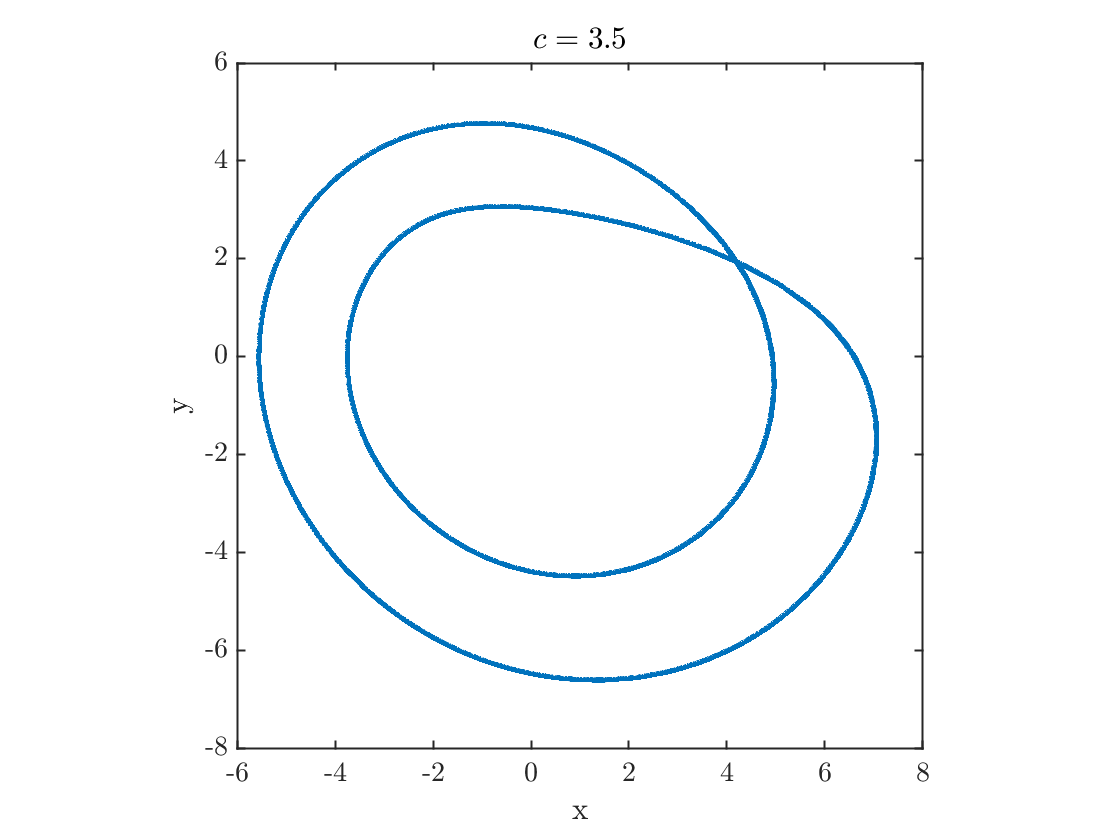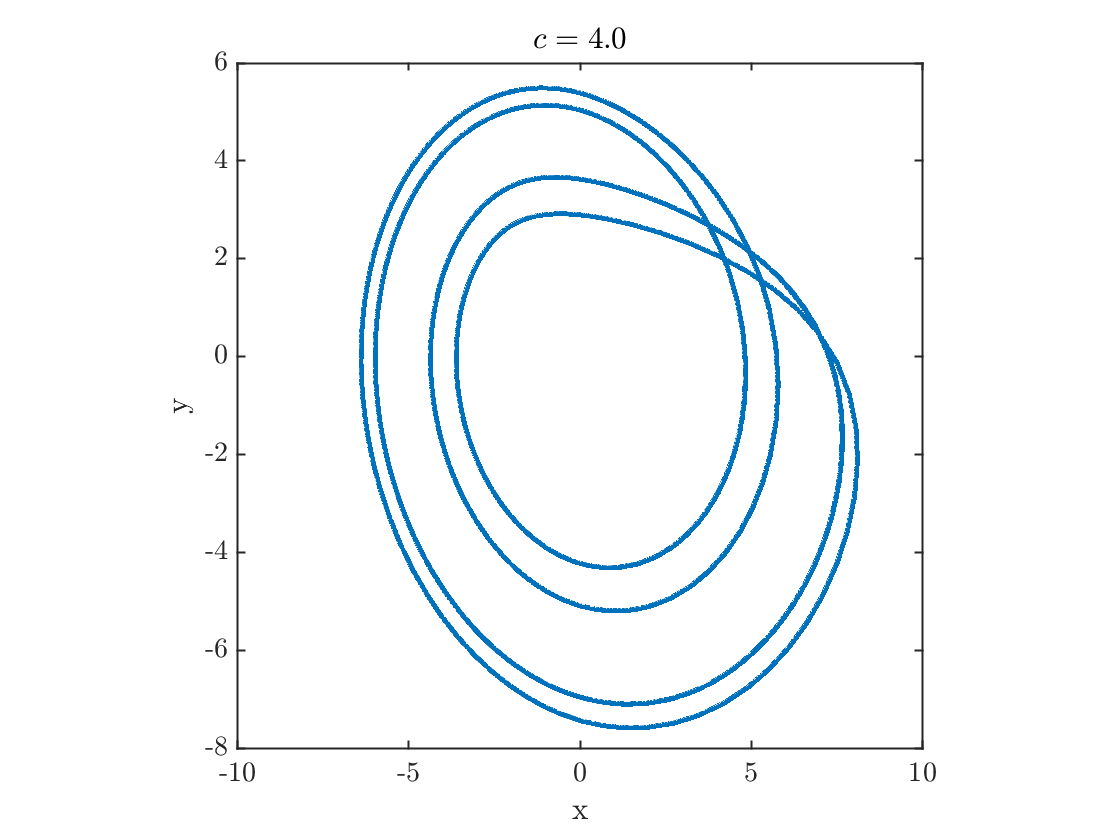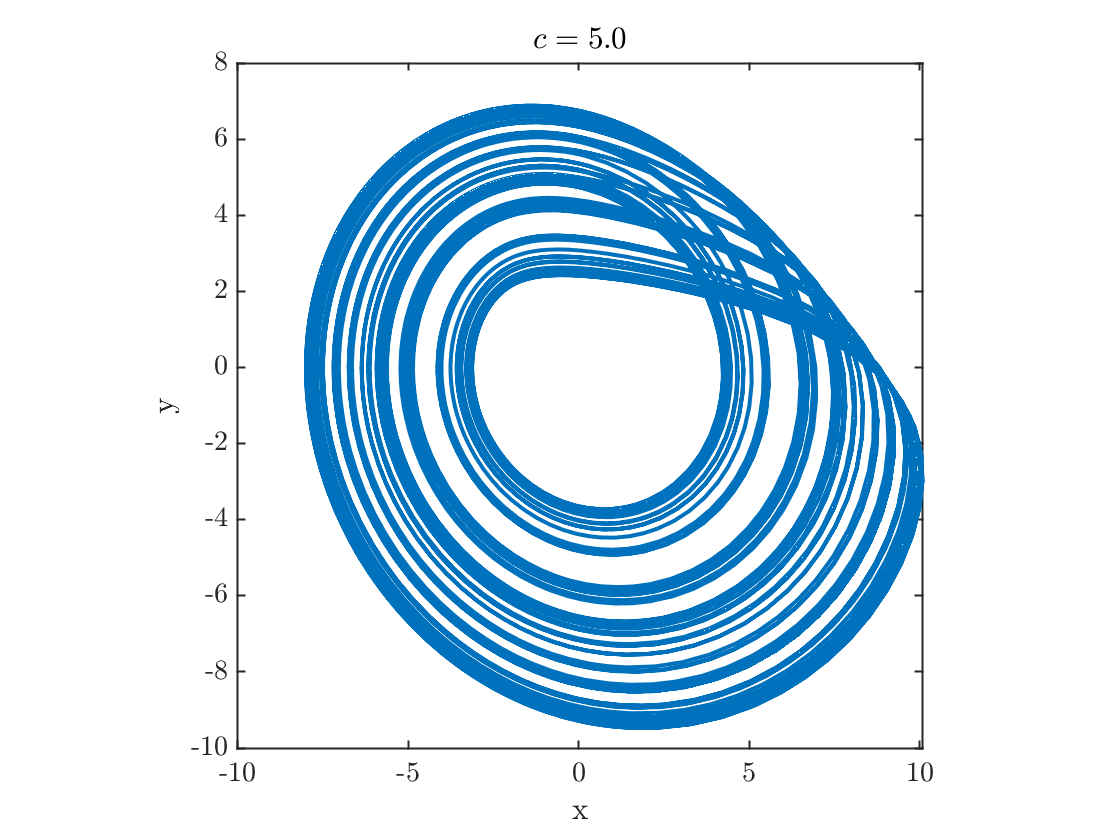## Computing Poincare Sections and Other Discrete Visualizations

A standard visualization is to plot the solution at a discrete series of times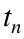where some event occurs. In a Poincaré section, we usually choose that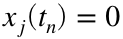and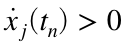(to ensure that the solution passes through the surface of section in the same direction each time). Figure 10.6.7 on p. 385 shows a slightly different section. The timesrepresent the successive maxima. Therefore we want to find timesfor which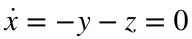(critical point condition) and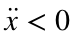.
MATLAB's ode solvers have an event-finding feature that allows us to locate the times at which events occur. Complete documentation can be found in the documentation or at this link. First, we need to create an events function, given below in the external functions section of this live script.
This function must return three vectors, each of the same length:
• value: each element of this vector is a function value whose zero defines an event
• isterminal: 1 if the associated event is terminal, that is, if the ode solver should quit when the event is located, 0 otherwise
• direction: describes the direction of the zero, that is whether the zero occurs while the function is increasing (direction=1), decreasing (direction=-1), or if either direction counts (direction=0)
Here is an example where we call the events finder
a=0.2; b=0.2; c=5;
myODE=@(t,y)rossler(t,y,a,b,c);
tspan=[0 100]; % Time span
y0=[1 0 0];
options=odeset('events',@rosslerXMaxEvent);
[t,y,te,ye,ie]=ode45(myODE,tspan,y0,options);
plot(t,y,te,ye(:,1),'o');
legend('x(t)','y(t)','z(t)','$x_n$');xlabel('t')